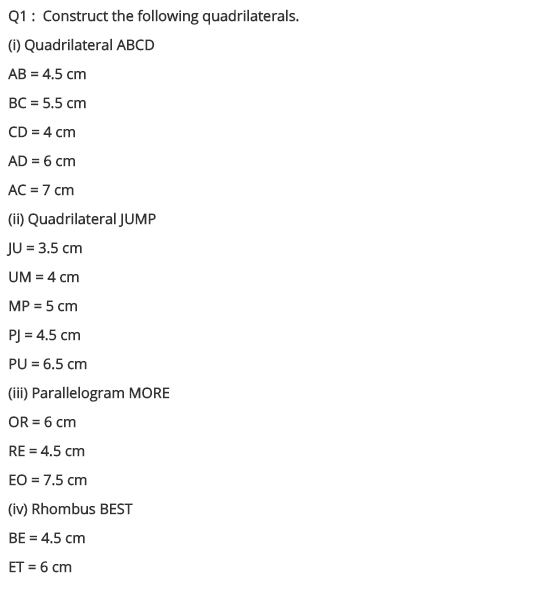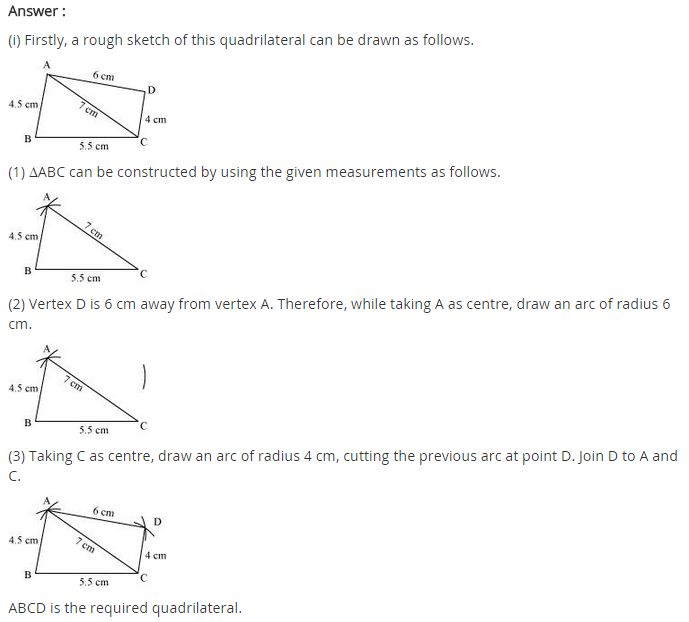# NCERT Solutions for Class 8 Maths Chapter 4 Practical Geometry

NCERT Solutions for Class 8 Maths Chapter 4 Practical Geometry

### NCERT Solutions for Class 8 Maths Chapter 4 Practical Geometry Exercise 4.1

Ex 4.1 Class 8 Maths Question 1.
AB = 4.5 cm, BC = 5.5 cm, CD = 4 cm, AD = 6 cm, AC = 7 cm
JU = 3.5 cm, UM = 4 cm, MP = 5 cm, PJ = 4.5 cm, PU = 6.5 cm
(iii) Parallelogram MORE
OR = 6 cm, RE = 4.5 cm, EO = 7.5 cm
(iv) Rhombus BEST
BE = 4.5 cm, ET = 6 cm
Solution:
(i) We have to draw first rough sketch.Construction:
Step I: Draw AB = 4.5 cm
Step II: Draw an arc with centre B and radius 5.5 cm.
Step III: Draw another arc with centre A and radius 7 cm to meet the previous arc at C.
Step IV: Draw an arc with centre C and radius 4 cm.
Step V: Draw another arc with centre A and radius 6 cm to cut the former arc at D.
Step VI: Join BC, AC, CD and AD.
(ii) We have to draw the first rough sketch.Thus ABCD is the required quadrilateral.
Construction:
Step I: Draw JU = 3.5 cm.
Step II: Draw an arc with centre J and radius 4.5 cm.
Step III: Draw another arc with centre U and radius 6.5 cm to meet the previous arc at P.
Step IV: Join JP and UP.
Step V: Draw an arc with centre U and radius 4 cm.
Step VI: Draw another arc with centre P and radius 5 cm to meet the previous arc at M.
Step VII: Join UM and PM.
Thus, JUMP is the required quadrilateral.
(iii) We have to draw the first rough sketch.Construction: (Opposite sides of a parallelogram are equal)
Step I: Draw OR = 6 cm.
Step II: Draw an arc with centre R and radius 4.5 cm.
Step III: Draw another arc with centre O and radius 7.5 cm to meet the previous arc at E.
Step IV: Join RE and OE.
Step V: Draw an arc with centre E and radius 6 cm.
Step VI: Draw another arc with centre O and radius 4.5 cm to meet the former arc at M.
Step VII: Join EM and OM.
Thus, MORE is the required parallelogram.
(iv) We have to draw first rough sketch.Construction: (All sides of a rhombus are equal)
Step I: Draw BE = 4.5 cm
Step II: Draw an arc with centre B and radius 4.5 cm.
Step III: Draw another arc with centre E and radius 6 cm to meet the previous arc at T.
Step IV: Join BT and ET.
Step V: Draw two arcs with centres E and T with equal radii 4-5 cm to meet each other at S. .
Step VI: Join ES and TS.
Thus, BEST is the required rhombus.## SabDekho

The Complete Educational Website